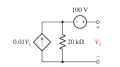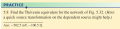# Thought process -- Thevenin problem

#### Doug Edmunds

Joined May 29, 2019
5This is an Practice example from the Hayt/Kemmerley/Durbin Engineering Circuit Analysis. They ask to find the Thevenin equivalent and give answers of -502.5 mV and -100.5 Ohms.I am trying to figure out the steps (thought process) to solve this problem with no success. Can someone walk through the steps they took to get this answer? BTW, this is self-study, not homework.

#### RBR1317

Joined Nov 13, 2010
668
But what could be simpler than Open-Circuit-Voltage & Short-Circuit-Current?

In the open circuit condition (V1=Voc) all the current flows through the resistor, so the voltage across the resistor is Voc-100 and the current through the resistor is 0.01·Voc amps. Then 0.01·Voc·20kΩ = Voc-100; Voc = -100/199

In the short circuit condition (V1=0) the current source supplies no current (because V1=0) so the current is Isc = 100V/20kΩ = 5 mA.

#### WBahn

Joined Mar 31, 2012
26,398
View attachment 185189

This is an Practice example from the Hayt/Kemmerley/Durbin Engineering Circuit Analysis. They ask to find the Thevenin equivalent and give answers of -502.5 mV and -100.5 Ohms.

View attachment 185190

I am trying to figure out the steps (thought process) to solve this problem with no success. Can someone walk through the steps they took to get this answer? BTW, this is self-study, not homework.
Don't make the problems harder than they are. Forget Thevenin, it is simply a circuit that needs to be analyzed.

It might help to put a box across the terminals so that it looks like the type of circuit your used to working with.

Now analyze it using either mesh current analysis or nodal analysis. Find the open circuit voltage by setting the right hand mesh current to zero and find the short circuit current by setting V1 to zero.